# Peak

Uphill leads 2 paths and 1 lift.

a) How many options back and forth are there?
b) How many options to get there and back by not same path are there?
c) How many options back and forth are there that we go at least once a lift?

Correct result:

a) n =  9
b) n =  6
c) n =  5

#### Solution:

$n_a = (2+1)\cdot (2+1) = 9$
$n_b = (2+1) \cdot 2 = 6$
$n_b = 2 \cdot 1 + 1 \cdot 2 + 1\cdot 1 = 5$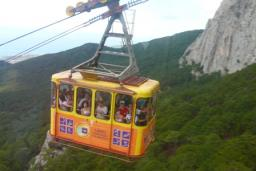We would be pleased if you find an error in the word problem, spelling mistakes, or inaccuracies and send it to us. Thank you!Tips to related online calculators
Would you like to compute count of combinations?

## Next similar math problems:

• Phone numbersHow many 7-digit telephone numbers can be compiled from the digits 0,1,2,..,8,9 that no digit is repeated?
• Boys and girlsThere are eight boys and nine girls in the class. There were six children on the trip from this class. What is the probability that left a) only boys b) just two boys
• Math logicThere are 20 children in the group, each two children have a different name. Alena and John are among them. How many ways can we choose 8 children to be among the selected A) was John B) was John and Alena C) at least one was Alena, John D) maximum one wa
• DivideHow many different ways can three people divide 7 pears and 5 apples?
• Stacks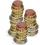Annie has a total of \$ 702. The money must be divided into stacks so that each buyer has the same amounth of dollars. How many options she have?
• Chess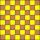How many ways can select 4 fields on classic chess board with 64 fields, so that fields don't has the same color?
• ClassroomOf the 26 pupils in the classroom, 12 boys and 14 girls, four representatives are picked to the odds of being: a) all the girls b) three girls and one boy c) there will be at least two boys
• STRESSED wordEach letter in STRESSED is printed on identical cards, one letter per card and assembled in random order. Calculate the probability that the cards spell DESSERTS when assembled.
• Flags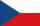How many different flags can be made from colors red, yellow, blue, green, white so that each flag consisted of three different colors?
• Chambers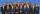The decision-making committee consists of three people. In order for the commission's decision to be valid, at least two members must vote in the same way. It is not possible not to vote in the commission, everyone only votes yes or no. We assume that the
• Neighborhood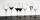I have 7 cups: 1 2 3 4 5 6 7. How many opportunities of standings cups are there if 1 and 2 are always neighborhood?There are 15 boys and 12 girls at the graduation party. Determine how many four couples can be selected.There are 20 boys and 10 girls in the class. How many different dance pairs can we make of them?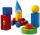3 children pulled 12 different toys from a box. Many ways can be divided toys so that each children had at least one toy?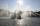The city has 7 fountains. Works only 6. How many options are there that can squirt ?How many ways can we divide nine workers into three workplaces if they need four workers in the first workplace, 3 in the second workplace and 2 in the third?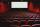5 friends went to the cinema. How many possible ways can sit in a row, if one of them wants to sit in the middle and the remaining's place does not matter?